# Graphs Dealing with Sine and Cosine Answer the

• Slides: 15
Download presentation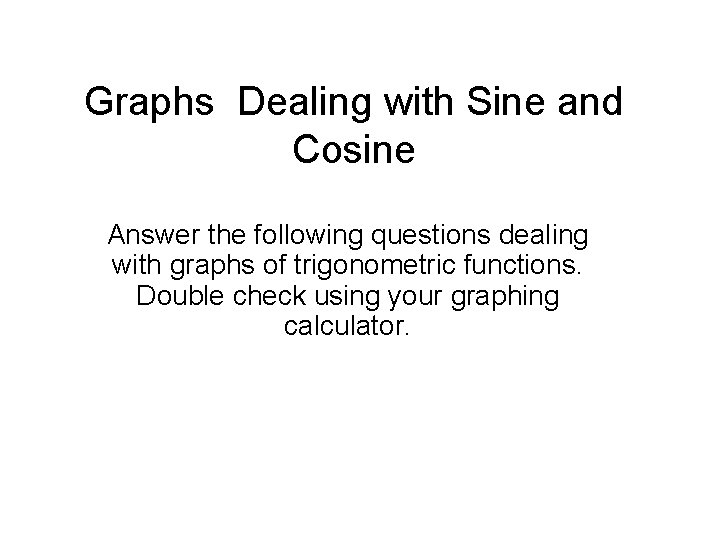Graphs Dealing with Sine and Cosine Answer the following questions dealing with graphs of trigonometric functions. Double check using your graphing calculator.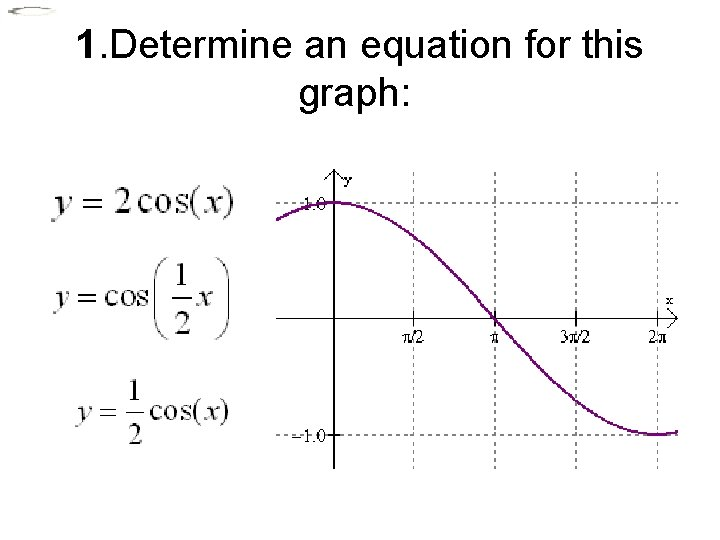1. Determine an equation for this graph: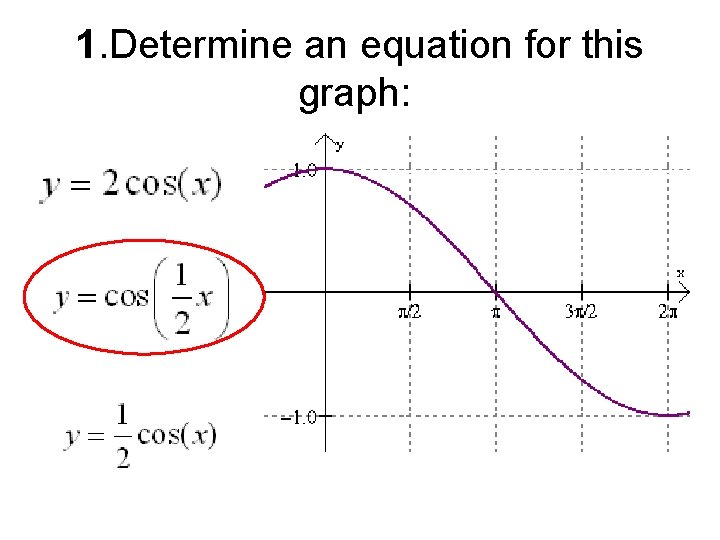1. Determine an equation for this graph:2. Determine an equation for this graph: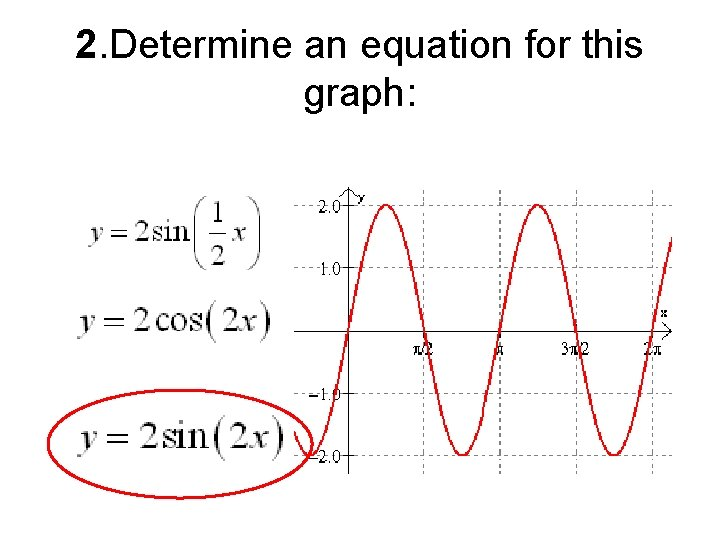2. Determine an equation for this graph: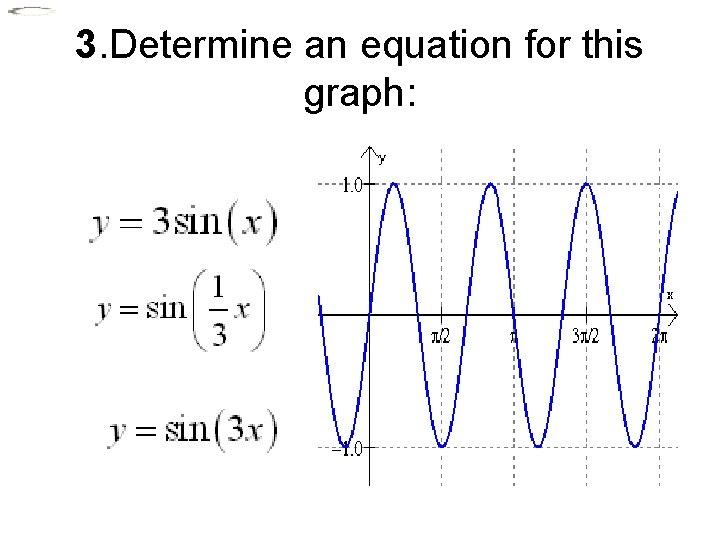3. Determine an equation for this graph: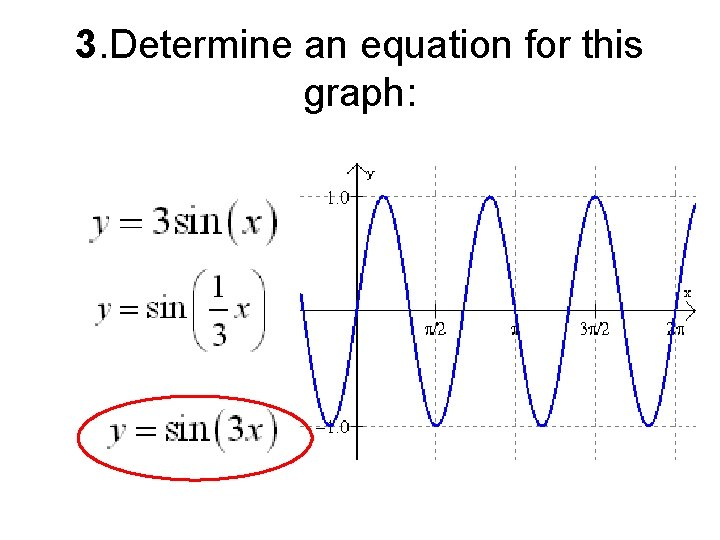3. Determine an equation for this graph: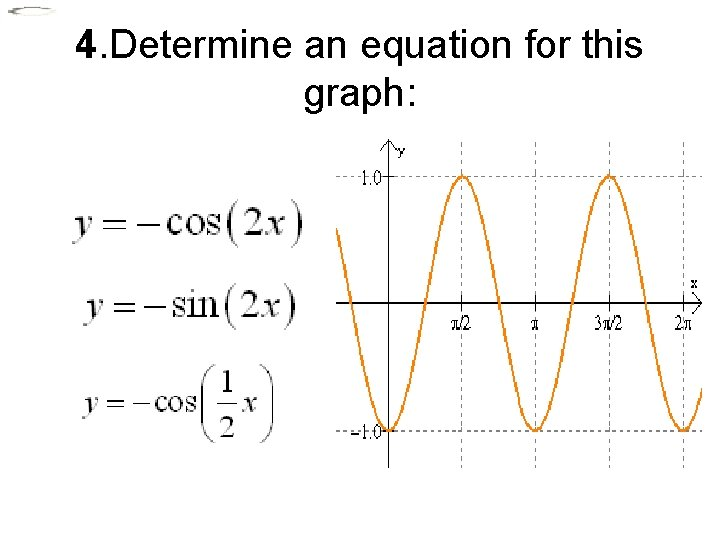4. Determine an equation for this graph:4. Determine an equation for this graph: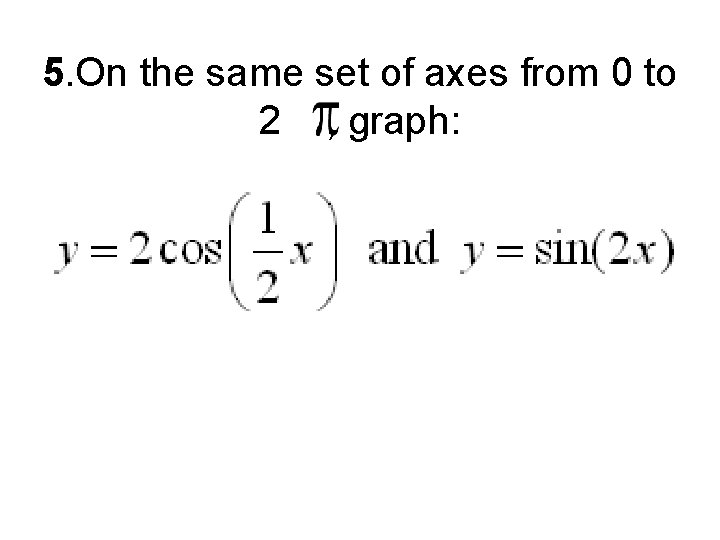5. On the same set of axes from 0 to 2 , graph: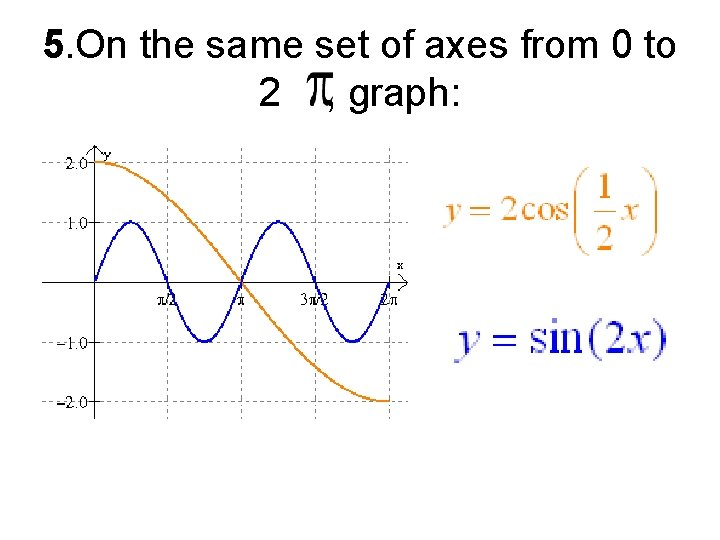5. On the same set of axes from 0 to 2 , graph: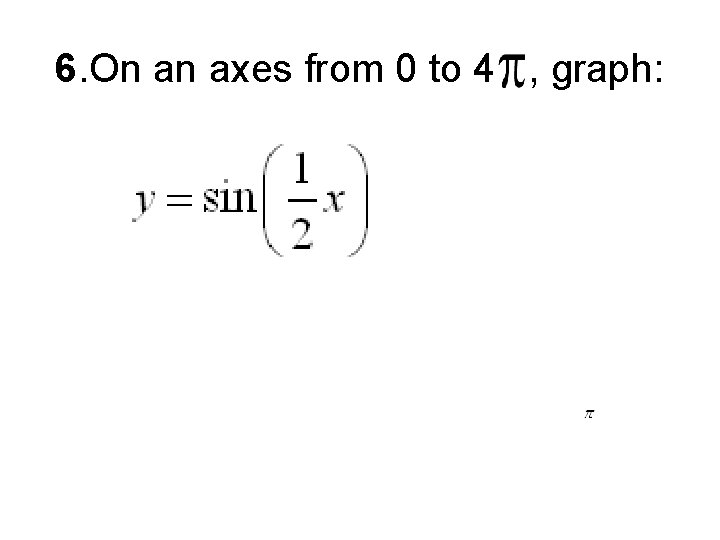6. On an axes from 0 to 4 , graph: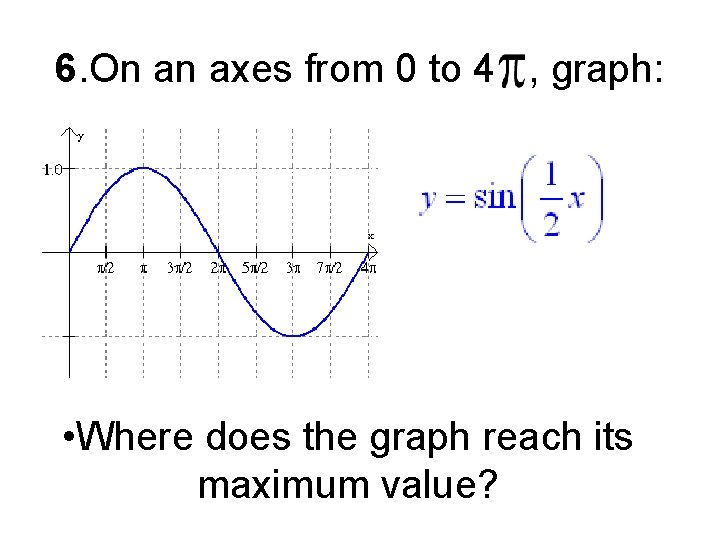6. On an axes from 0 to 4 , graph: • Where does the graph reach its maximum value?7. On the same set of axes from 0 to 2 , graph: • How many points satisfy the equation ? ? ? ?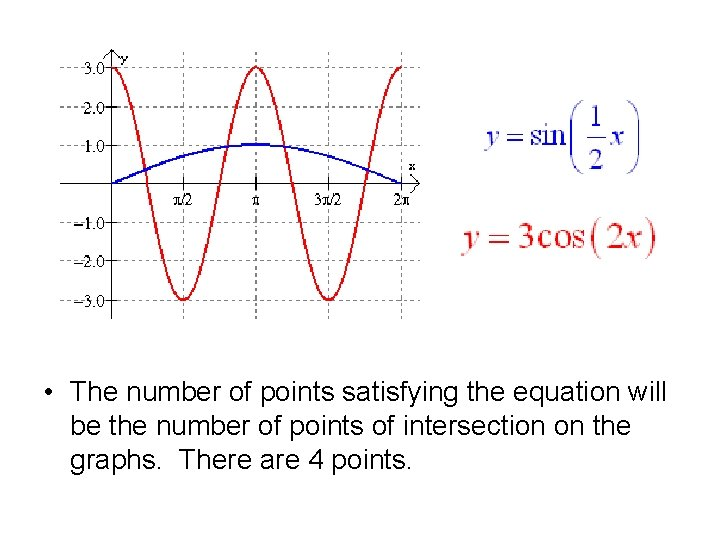• The number of points satisfying the equation will be the number of points of intersection on the graphs. There are 4 points.